# A Tip A Day — Python Tip #8: Why should we Normalize image pixel values or divide by 255? | Dev Skrol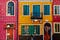Photo by Hari Nandakumar on Unsplash

In our previous post A Tip A Day — Python Tip #7: OpenCV — CV2: imread() and resize(), we have explored a simple image and its pixel values.
The pixel values can range from 0 to 256. Each number represents a color code.
When using the image as it is and passing through a Deep Neural Network, the computation of high numeric values may become more complex.
To reduce this we can normalize the values to range from 0 to 1.

In this way, the numbers will be small and the computation becomes easier and faster.
As the pixel values range…

# Python Tip #7: OpenCV — CV2: imread() and resize() | Dev Skrol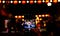Photo by Hari Nandakumar on Unsplash

In this article we are going to learn some of the major use of OpenCV or CV2.

OpenCV is a library mainly used to read/write and manipulate images.

You can find the official page here https://pypi.org/project/opencv-python/.

Installation: pip install opencv-python

Importing Library: import cv2

Supported Versions: 3.6, 3.7, 3.8, 3.9

# Basic understanding of image:

We all know that an image is nothing but an array of pixels.
Each pixel is a number.

Based on the number the color of the pixel will be.

Example: 0 — Black and 1 — White.

# Read Image: cv2.imread()

Lets consider we have a small image of 28 X 28 pixels.

`import…`

# Deep Learning — Classification Example

Hello Everyone!!! Its an immense pleasure to write today as this is the first post I am able to write in 2021. Happy New Year!!! 🥳 🎂 🎉

In this article we are going to see the continuation of Deep Learning techniques. We are going to see an Deep Learning model with a Classification Example.

In our last article, we learned about use a simple Neural Network to solve regression problems — Artificial Neural Network Explained with an Regression Example.

If you missed the prequels, please check below:

Artificial Intelligence — A brief Introduction and history of Deep Learning

How…

# Artificial Neural Network Explained with an Regression Example

In this article, we are going to discuss about the basics of Implementing a simple Artificial Neural Network (ANN).

It is recommended to know how Artificial Neurons mimic Neurons of human brain. Please read this article for easy understanding. If you are excited to know about the history of ANN, please check this article.

# Architecture Of ANN:

The architecture of ANN contains 4 main components.

Input Layer receives input:

Input layer is a set of nodes which takes the input data as one per node. …

# Python Tip #6 — Pandas Merge

Pandas concat & append works like an array concatenation either column wise or row wise.

But Merge & Join works similar to Database table joins. Lets see Merge in this article and learn about Join in next post!

So, Merge can be used when we need to join two data frames having different columns and there is a key column in both the data frames.

For example, if we have two data frames, one with store details and another with product details of all the stores, we can merge the data frames using shop Id.

Signature:
pd.merge(left,
right,
how: str = ‘inner’,
on=None,
left_on=None,
right_on=None,
left_index: bool…

# Python Tips — Pandas Concat & Append

In this article we are going to learn about Concat & Append and the comparision between these two functions.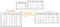Concat Vs Append — Image by Author
`import pandas as pd`

Lets take 2 dataframes of fruits.

`fruit = { 'orange' : [3,2,0,1], 'apple' : [0,3,7,2], 'grapes' : [7,14,6,15] } df1 = pd.DataFrame(fruit) df1`

Output: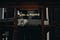Photo by Gaelle Marcel on Unsplash

# Python Tips — Show Progress Bar using TQDM

Have you ever wished to see a progress bar in Jupyter Notebook when executing a complex function in for loop?

Its possible in Python.

There is a package tqdm which allows us to show progress bar.
Also it is simple to use!!!

While implementing a for loop wrap around the iterable object using the function tqdm to show the progress of the for loop execution.

Lets see how to do it.

As we are going to use a simple for loop of 10 numbers using range(), lets use a sleep method to slower the process. …

# Python Tips — Pandas Apply Function

Apply function takes a function as an argument and execute the function in all the elements of the dataframe.

For example, if we want to create a new column which is the square root of another column’s values or apply a complex function and combine one or more columns or when creating new features using the existing features for feature engineering.

Syntax: df.apply(func, axis=0, broadcast=None, raw=False, reduce=None, result_type=None, args=(), **kwds)

df — a pandas series.

func — A lambda function or a normal function

axis — 0 — rows which is default, 1 — columns

`import pandas as pd import…`

# Python Tips — iloc & loc vs iloc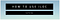Image by Author

Today we are going to learn how to use iloc to get values from Pandas DataFrame and we are going to compare iloc with loc.

To learn about loc, please check A tip A day — Python Tip #1 — loc.

# Using iloc:

• iLoc uses only numbers/indexes (strictly numerical values) to get values from a Pandas DataFrame.
• Apart from numerical values, it accepts boolean arrays similar to Loc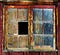Source: Photo by Nate Bell on Unsplash

# Best way to Impute categorical data using Groupby — Mean & Mode

We know that we can replace the nan values with mean or median using fillna(). What if the NAN data is correlated to another categorical column?

What if the expected NAN value is a categorical value?

Below are some useful tips to handle NAN values.

Definitely you are doing it with Pandas and Numpy.

`import pandas as pdimport numpy as np`

# ngroup

`cl = pd.DataFrame({'team':['A','A','A','A','A','B','B','B','B','B'],                   'class'['I','I','I','I','I','I','I','II','II','II'],'value': [1, np.nan, 2, 2, 3, 1, 3, np.nan, 3,1]})`## Asha Ponraj

Data Science & Machine Learning Enthusiast | Software Developer | Blog Writer | https://devskrol.com/ | www.linkedin.com/in/asha-ponraj-a5a76b50